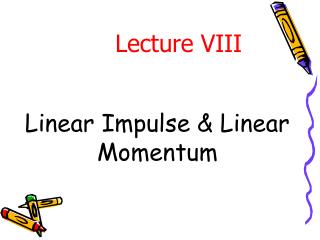DownloadDownload PresentationLinear Impulse & Linear Momentum

# Linear Impulse & Linear Momentum

Télécharger la présentation## Linear Impulse & Linear Momentum

- - - - - - - - - - - - - - - - - - - - - - - - - - - E N D - - - - - - - - - - - - - - - - - - - - - - - - - - -
##### Presentation Transcript

1. Linear Impulse & Linear Momentum Lecture VIII

2. Introduction • From Newton’s 2nd Law: SF=m a =mv.= d/dt (mv) • The term mv is known as the linear momentum, and it is abbreviated by G. SF=G.(1) This formula states that the resultant of all forces acting on a particle equals its time rate of change of linear momentum. • The SI unit of the linear momentum is N.s. • Note that the rate of change of linear momentum G. is a vector quantity, and its direction coincides with the direction of the resultant forces, i.e. coincides with the direction of the acceleration. Where the direction of G coincides with the direction of the velocity. • In rectangular coordinates, the three scalar components of formula (1) are: SFx =Gx. SFy =Gy. SFz =Gz. Note: the momentum formula is valid as long as the mass m is not changing.

3. The Linear Impulse-Momentum Principle Force, F F2 • From formula (1), • SF =G. SF =d/dt (G) SFdt =d (G) • By the integration of both sides, • The product of force and time, represented by the term , is known as the linear impulse. • The formula (2) states that the total linear impulse on m equals to the change in the linear momentum of m. • In rectangular coordinates, the magnitudes versions of (2) are: F1 t1 Time, t t2 Note: If the force, which is acting on a particle, varies with time, the impulse will be the integration of this force w.r.t. time or the area under the above curve. or (2) Conservation of Linear Momentum When the sum of all forces acting on a particle is zero, i.e SF = 0, thus, DG = 0 or G1 = G2

4. Impact • Impact: is the collision between two bodies. It leads to the generation of relatively large contact forces that act over a very short interval of time. There are two main types of impact, they are: a) direct central impact and b) oblique central impact. • a) Direct central impact: a collinear motion of two spheres of masses m1 and m2, traveling with velocities v1 and v2. If v1 > v2, collision will occur with contact forces directed along the line of centers of the two spheres. Note that the sum of forces are zero or relatively small (produces negligible impulses), thus, F1 F2

5. Impact – Cont. • Coefficient of restitution (e): in the direct impact formula, there are two unknowns . An additional relationship is needed to find the these final velocities. This relationship must reflect the capacity of the contacting bodies to recover from the impact and can be expressed in by the ratio e of the magnitude of the restoration impulse to the magnitude of the deformation impulse. This ratio is known as the coefficient of restitution. For particle 1 By eliminating vo in both equation For particle 2

6. Impact – Cont. • b) Oblique central impact: the initial and final velocities are not parallel. The directions of the velocity vectors are measured from the direction tangent to the contacting surfaces. • However, there are four unknowns; they are To find them, the following four formulas will be used: (1) (4) (2) Note: There is no impulse on either particle in the t-direction (3)

7. Linear Impulse & Linear MomentumExercises

8. Exercise # 1 3/179: A 75-g projectile traveling at 600 m/s strikes and becomes embedded in the 50-kg block, which is initially stationary. Compute the energy lost during the impact. Express your answer as an absolute value |DE| and as a percentage n of the original system energy E.

9. 3/180: A jet-propelled airplane with a mass of 10 Mg is flying horizontally at a constant speed of 1000 km/h under the action of the engine thrust T and the equal and opposite air resistance R. The pilot ignites two rocket-assist units, each of which develops a forward thrust T0of 8 kN for 9 s. If the velocity of the airplane in its horizontal flight is 1050 km/h at the end of the 9 s, calculate the time-average increase DR in air resistance. The mass of the rocket fuel used is negligible compared with that of the airplane . Exercise # 2

10. Exercise # 3 3/199: Car B (1500 kg) traveling west at 48 km/h collides with car A (1600 kg) traveling north at 32 km/h as shown. If the two cars become entangled and move together as a unit after the crash, compute the magnitude v of their common velocity immediately after the impact and the angle qmade by the velocity vector with the north direction .

11. Exercise # 4 3/248: The sphere of mass m1travels with an initial velocity v1directed as shown and strikes the sphere of mass m2. For a given coefficient of restitution e, determine the mass ratio m1/m2which results in m1being motionless after the impact.

12. Exercise # 5 3/254: The steel ball strikes the heavy steel plate with a velocity vo= 24 m/s at an angle of 60° with the horizontal. If the coefficient of restitution is e = 0.8, compute the velocity v and its direction qwith which the ball rebounds from the plate.